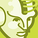# New to Qlik Sense

If you’re new to Qlik Sense, start with this Discussion Board and get up-to-speed quickly.

Announcements
Don't miss the upcoming Q&A with Qlik session on Qlik Application Automation on November 16th! REGISTER NOW
cancel
Showing results for
Did you mean:Contributor III

## QLIK server RAM usage - data caculation

Hello, I am trying to fix performance problems with Qlik sense server. Qlik is installed in virtual workstation with dedicated memory of 4GB ( some of that will use the operating system so we have about 3GB of memory for Qlik Sense calculation. - for 5 users ) As Qlik apps are getting bigger and more complex the memory usage is going up to 100%. I think that main problem is the growing volume of data being loaded to Qlik. Is there any way how to measure or calculate the volume of data that i am loading from remote SQL servers to Qlik in the virtual machine ?

Labels (7)

• ### SQL

1 Solution

Accepted SolutionsPartner

RAMInitial =  SizeOnDisk ×  FileSizeMultiplier ; this is the initial RAM footprint for any application

FileSizeMultiplier: range between 2-10 (it's a compression ratio depending on the data contained in the app)

RAMperUser = RAMinitial ×  userRAMratio ; this is the RAM per each incremental user

userRAMratio: range between 1% -10%

Total RAM used per app :

TotalRAM = (RAMperUser × No. users) + RAMinitial

Example:

SizeOnDisk = 1 GB

File Size Multiplier 6 (range is from 2 to 10)

RAMinitial = 1 * 6 = 6 GB

If we take a RAM Ratio per User of 6% then it is 0.06 (range is from 1 to 10)

RAMperUser = 6 x 0.06 = 0.36GB

Then RAM Required for 30 Users

TotalRAM  =  (RAMperUser × No. users) + RAMinitial
TotalRAM  =  (0.36 * 30) + 6
TotalRAM  = 16.8 GB

found here

https://support.qlik.com/articles/000058871

Regards

2 RepliesPartner

RAMInitial =  SizeOnDisk ×  FileSizeMultiplier ; this is the initial RAM footprint for any application

FileSizeMultiplier: range between 2-10 (it's a compression ratio depending on the data contained in the app)

RAMperUser = RAMinitial ×  userRAMratio ; this is the RAM per each incremental user

userRAMratio: range between 1% -10%

Total RAM used per app :

TotalRAM = (RAMperUser × No. users) + RAMinitial

Example:

SizeOnDisk = 1 GB

File Size Multiplier 6 (range is from 2 to 10)

RAMinitial = 1 * 6 = 6 GB

If we take a RAM Ratio per User of 6% then it is 0.06 (range is from 1 to 10)

RAMperUser = 6 x 0.06 = 0.36GB

Then RAM Required for 30 Users

TotalRAM  =  (RAMperUser × No. users) + RAMinitial
TotalRAM  =  (0.36 * 30) + 6
TotalRAM  = 16.8 GB

found here

https://support.qlik.com/articles/000058871

RegardsContributor

You can even look for optimizing the application by following best practices and removing unused fields, expressions etc. Which can reduce the size of the app and inturn help in reduced RAM utilization.